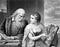# Expectation & Variance of OLS Estimates

In one of my previous articles, I had derived the OLS estimates for simple linear regression. I’ll try to dig a little deeper and explain some more features of these estimates.

As shown earlier, a simple regression model is expressed as:

Here α and β are the regression coefficients i.e. the parameters that need to be calculated to understand the relation between Y and X. i has been subscripted along with X and Y to indicate that we are referring to a particular observation, a particular value associated with X and Y. …

# Introduction

Through this article, I am delighted to put forward a parallelism that enraptured my curious intellect. Blending scientific and economic theories is my passion. I think about a scientific topic, a law or a formula and try to use it as a foundation for an economic ideology. It was during this thought process, that I discovered the correlation. I have also tried to use this correlation to answer some of the most fundamental questions in economics. So, let’s start.

Chemical kinetics is the branch of chemistry that deals with rates of chemical reactions. A chemical reaction is essentially a chemical…

# Simplifying Multivariate Regressions using Matrices

What is a Multivariate Regression?

Multivariate regression is a tool used by statisticians to express the relationship between a dependent variable (Yᵢ) and several independent variables (X₁ᵢ, X₂ᵢ, X₃ᵢ, …. Xₖᵢ). In general, multivariate regression models can be represented as:

Yᵢ is called the regressand and Xᵢ‘s are called regressors. εᵢ is the associated error, a term used to quantify the effect of unobserved factors in the regression. The β‘s represent regression coefficients i.e. the parameters which will help us in understanding the relationship between Y and X’s. k represents the number of regressors or dependent variables in our regression…

# The Simple Regression:

In Econometrics, a simple regression is a tool used to establish a relationship between 2 variables. One of the variables (Y) is called the dependent variable or the regressand, while the other variable (X) is called the independent variable or the regressor. Mathematically, a simple regression model is expressed as:

Here α and β are the regression coefficients i.e. the parameters that need to be calculated to understand the relation between Y and X. i has been subscripted along with X and Y to indicate that we are referring to a particular observation, a particular value associated with X and…

# Le Chatelier’s Principle and the Invisible Hand

In his prominent and august book, ‘The Theory of Moral Sentiments’, published in 1759, renowned economist Adam Smith first introduced the concept of the ‘Invisible Hand’. The invisible hand is often used as a metaphor to describe the unobservable force that facilitates the self-adjustment mechanisms of markets, allowing them to reach the stage of equilibrium. Such an equilibrium can be described in many ways- using microeconomic models of demand and supply, macroeconomic models of aggregate demand and aggregate supply et al. The philosophical model is also used to describe how individual pursuits of self-interests can result in unintended benefits accruing…

# God, Life, and EconomicsAn engraving by W. Ridgway (published in 1878) after Daniel Huntington’s 1868 painting Philosophy and Christian Art

As I envisioned my first Economics lecture at my dream college, I wondered about the first question my professor would ask us students. She would probably begin by asking “What is Economics?”. I imagined each one giving their own definition. I could recall the various definitions I had read in my textbooks. None of them seemed to do justice to this elephantine subject. It was then that I drew an interesting correlation between God, Life, and Economics.

How would you define economics?

You might say, “Economics is the agent that ties the relationship between unlimited human wants and scarce resources…## Naman Agrawal

Economics Enthusiast